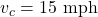## A boat travels upstream for 360 miles in 4 hours and returns in 3 hours traveling downstream in a local stream of water. What is the rate of

Question

A boat travels upstream for 360 miles in 4 hours and returns in 3 hours traveling downstream in a local stream of water. What is the rate of the boat in still water and the rate of the current?

in progress 0
3 weeks 2021-08-23T23:36:54+00:00 1 Answers 2 views 0

Rate of boat in still water = 105 mph

Rate of current = 15 mph

Explanation:

Let the speed of the boat in still water be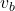.

Let the speed of the current be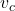.

When the boat goes upstream, it moves against the current. Hence, its velocity will be relative to that of the current. This is given by 360/4 = 90 mph.

This relative velocity is the difference between the speed of the boat in still water and that of the current: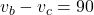In the downstream, the boat moves with the current. The resultant velocity is the sum of the velocities of boat in still water and current.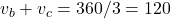Solving both equations simultaneously by elimination method,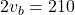(adding both equations)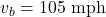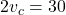(subtracting the first from the second equation)